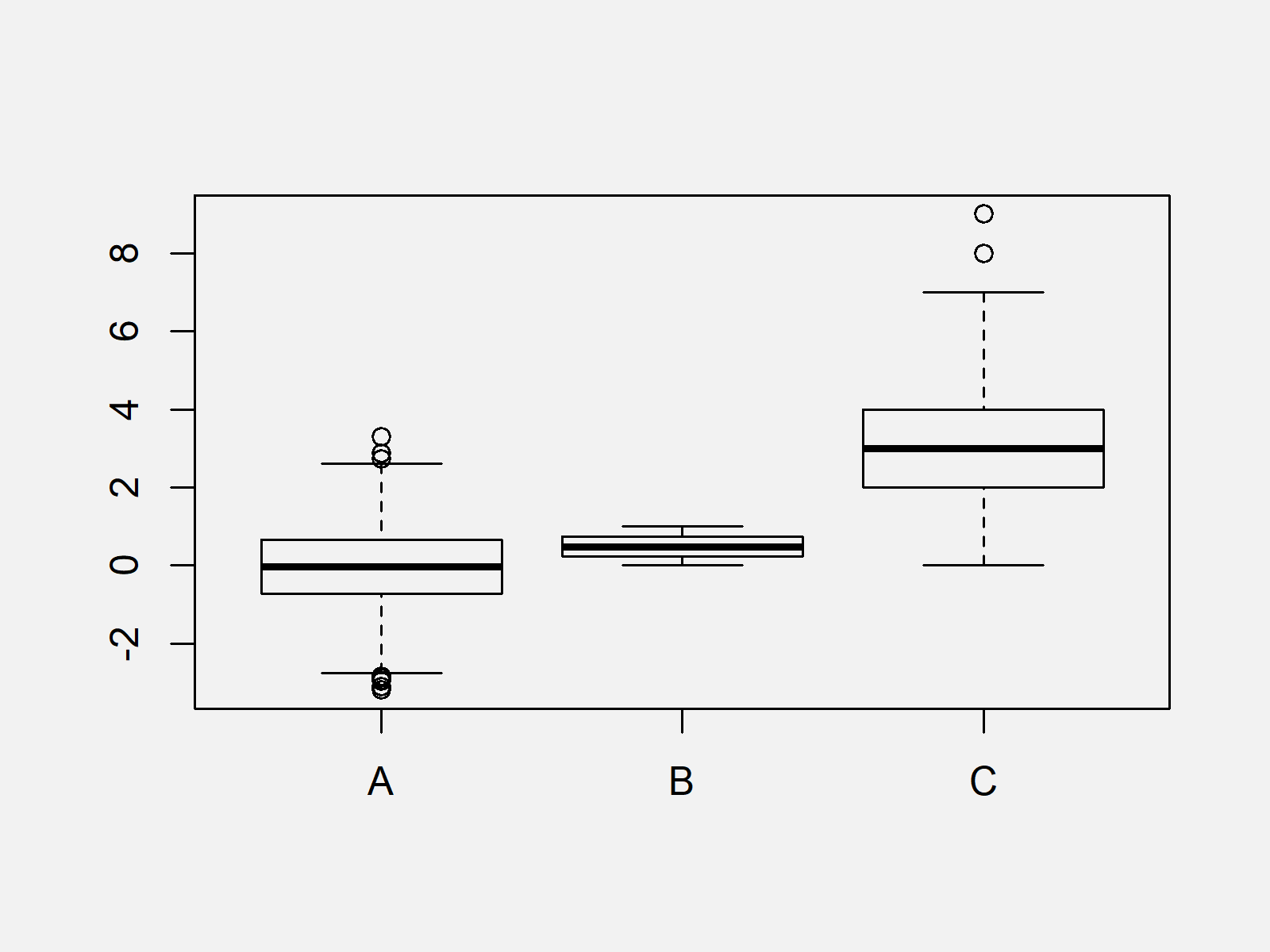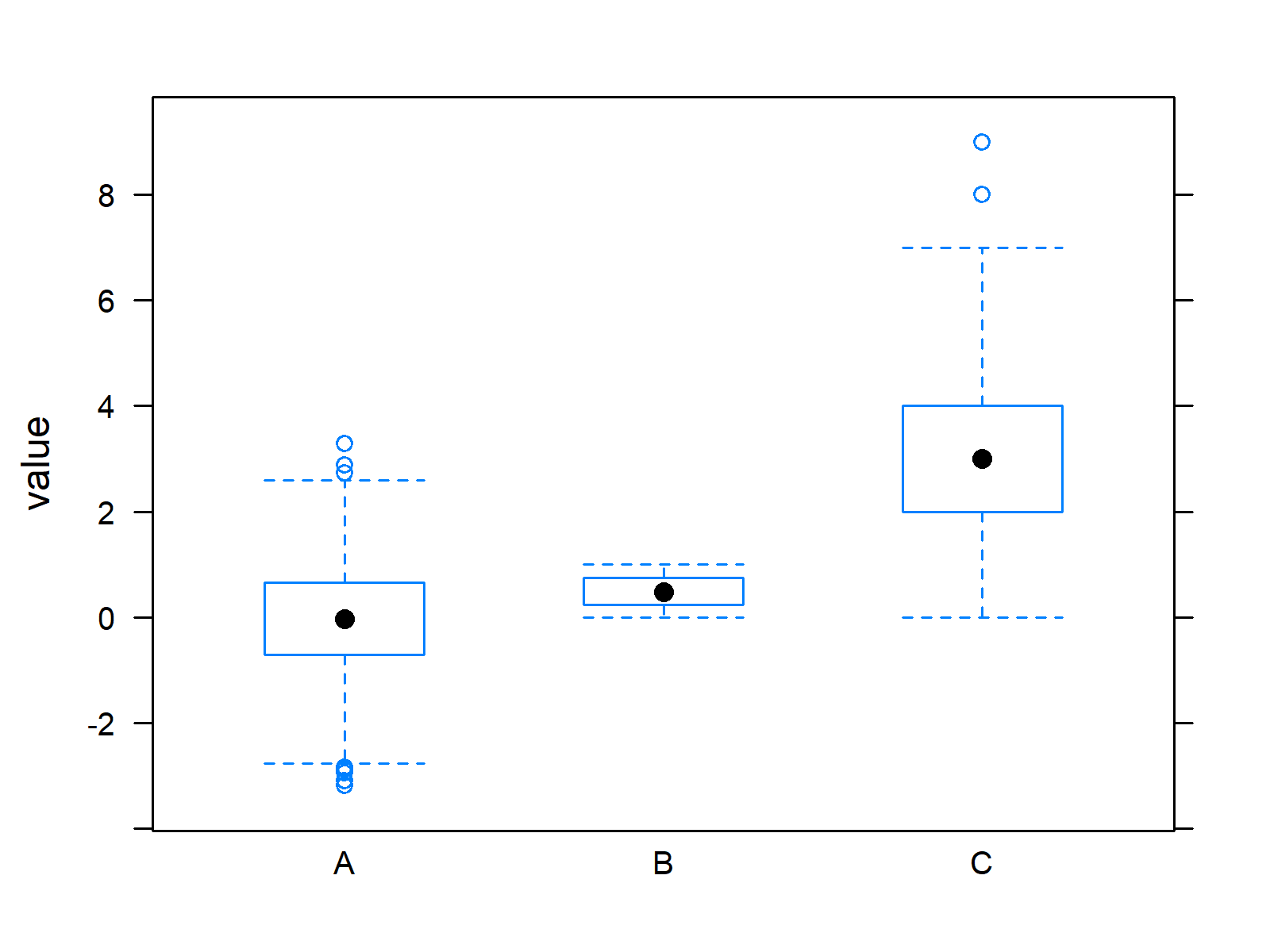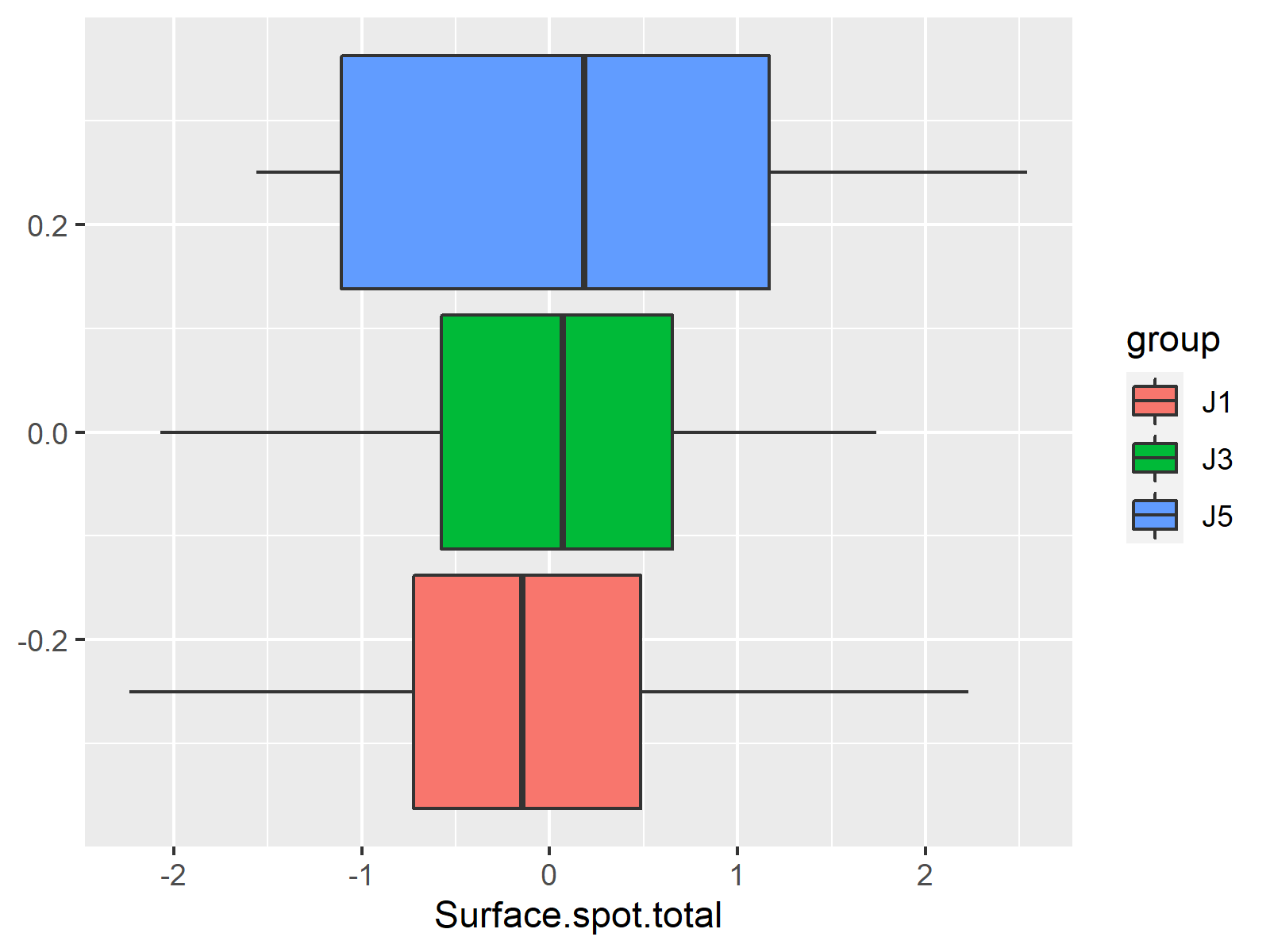# Draw Multiple Boxplots in One Graph in R Side-by-Side (4 Examples)

In this tutorial you’ll learn how to plot several boxplots side-by-side in the same graphic in the R programming language.

The article will contain these content blocks:

Let’s start right away!

## Creation of Example Data

First, we’ll need to create some data that we can use in the following examples:

```set.seed(75829547)                                           # Create example data
data <- data.frame(A = rnorm(1000),
B = runif(1000),
C = rpois(1000, 3))
#             A          B C
# 1  0.53802755 0.23042233 3
# 2 -0.81263292 0.03925386 3
# 3  0.15503948 0.37912312 3
# 4  0.73903916 0.51420032 3
# 5 -0.07919366 0.01956273 2
# 6 -1.56211181 0.92004033 2```

The previously shown output of the RStudio console shows the structure of our example data – It consists of three numeric columns A, B, and C. Each of these variables should be drawn as separate boxplot in the same graphic window in R.

## Example 1: Drawing Multiple Boxplots Using Base R Graphics

In Example 1, I’ll illustrate how to use the basic installation of the R programming language to plot several boxplots in the same graph. For this, we simply need to insert the name of our data frame into the boxplot function:

`boxplot(data)                                                # Applying boxplot function`As shown in Figure 1, we created a plot showing each of our variables as different boxplot with the previous syntax.

The previous R syntax is very simple. However, the output looks not really pretty yet. In the following examples I’ll therefore explain how to create more advanced boxplot graphics with the ggplot2 and lattice packages in R.

If you want to learn more about improving Base R boxplot graphics, you may have a look here.

## Example 2: Drawing Multiple Boxplots Using ggplot2 Package

In Example 2, I’ll show how to use the functions of the ggplot2 package to create a graphic consisting of multiple boxplots.

To draw such a plot with the ggplot2 package, we need data in long format and we can convert our example data to long format using the reshape package.

If we want to apply the functions of the reshape2 package, we first have to install and load reshape2:

```install.packages("reshape2")                                 # Install reshape2 package

Now, we can convert our data to long format using the melt function provided by the reshape2 package:

```data_long <- melt(data)                                      # Reshaping data frame
#   variable       value
# 1        A  0.53802755
# 2        A -0.81263292
# 3        A  0.15503948
# 4        A  0.73903916
# 5        A -0.07919366
# 6        A -1.56211181```

If we want to draw boxplots with the ggplot2 package, we also need to install and load ggplot2:

```install.packages("ggplot2")                                  # Install ggplot2 package

Finally, we can draw all boxplots to a ggplot2 graphic:

```ggplot(data_long, aes(x = variable, y = value)) +            # Applying ggplot function
geom_boxplot()```The output of the previously shown code is illustrated in Figure 2: A ggplot2 graph containing multiple boxplots side-by-side.

## Example 3: Drawing Multiple Boxplots Using lattice Package

Another popular package for drawing boxplots is the lattice package.

If we want to use the functions of the lattice package, we first need to install and load lattice:

```install.packages("lattice")                                  # Install lattice package

Now, we can apply the bwplot function to draw our boxplots. Note that we are using the long data frame that we have created in the previous example:

`bwplot(value ~ variable, data_long)                          # Applying bwplot function`As illustrated in Figure 3, we created a graphic with multiple boxplots with the previous code.

## Example 4: Drawing Multiple Boxplots for Each Group Side-by-Side

So far, we have drawn only one boxplot for each variable of our example data. However, it is possible to add another layer by drawing multiple boxplots for each group of a variable.

Let’s do this in R!

First, we need some example data:

```data(iris)                                                   # Loading iris flower data set
#   Sepal.Length Sepal.Width Petal.Length Petal.Width Species
# 1          5.1         3.5          1.4         0.2  setosa
# 2          4.9         3.0          1.4         0.2  setosa
# 3          4.7         3.2          1.3         0.2  setosa
# 4          4.6         3.1          1.5         0.2  setosa
# 5          5.0         3.6          1.4         0.2  setosa
# 6          5.4         3.9          1.7         0.4  setosa```

As you can see based on the previous output of the RStudio console, our example data is the popular iris flower data set which consists of four numeric variables and the Species grouping variable.

I’m going to use the ggplot2 package in this example. For that reason, we need to reshape the iris data frame from wide to long format:

```iris_long <- melt(iris, id = "Species")                      # Reshaping iris data
#   variable       value
# 1        A  0.53802755
# 2        A -0.81263292
# 3        A  0.15503948
# 4        A  0.73903916
# 5        A -0.07919366
# 6        A -1.56211181```

Now, we can draw our ggplot2 boxplot graph as shown below. Note that we are specifying the color argument to be equal to our grouping column Species:

```ggplot(iris_long, aes(x = variable, y = value, color = Species)) +  # ggplot function
geom_boxplot()```As shown in Figure 4, the previous R syntax created a graphic that shows a boxplot for each factor level group of each variable of our data frame.

## Video, Further Resources & Summary

Do you want to know more about boxplots in R? Then you may watch the following video of my YouTube channel. I explain the content of this tutorial in the video.

Please accept YouTube cookies to play this video. By accepting you will be accessing content from YouTube, a service provided by an external third party.If you accept this notice, your choice will be saved and the page will refresh.

In addition, you might want to have a look at the related articles on this homepage. A selection of interesting posts about graphics in R can be found below.

In summary: You learned in this article how to create a graph containing multiple boxplots with one or multiple factor labels in the R programming language. If you have additional questions, tell me about it in the comments section below.

Subscribe to the Statistics Globe Newsletter

•S
February 6, 2022 8:48 pm

Hi, in example #4 is there anyway to edit the category labels? (i.e. Sepal.Length to Sepal Length)

•Cassio
April 9, 2022 4:35 pm

Thank you Joachim for these useful and straightforward examples

•April 11, 2022 6:44 am

Hey Cassio,

Thanks a lot for the very kind feedback! Glad you like the tutorials.

Regards,
Joachim

•Aj Mota
April 15, 2022 3:23 pm

Thanks!!! Joachim,

•April 18, 2022 8:50 am

Thank you very much, glad it was useful! 🙂

•Nazeleternel
May 10, 2022 9:39 am

Hey, thank you for your tutorial, I have a question.
I’m trying to create multiple boxplots, but the number of lines for each boxplot isn’t the same.
Since I don’t have the same number of observations, I can’t create a data.frame to work with. Is there any way I could bypass this issue with R?

•May 10, 2022 10:19 am

Hey Nazeleternel,

Could you please share your code and illustrate the first few rows of your data, i.e. what is returned when you run head(your_data) ?

Regards,
Joachim

•Nazeleternel
May 10, 2022 11:23 am

Here is the kind of data I am using:

Label J Surface.de.la.coupe Surface.de.l.epithelium
4 PBS2-1-2_Stitch 3 364564058 12176663
5 PBSA3-1-1-0504_Stitch 3 401801863 12268656
6 PBSA4-1-2-0504_Stitch 3 325869468 13200887
Surface.des.spots.dans.la.coupe Somme.Intensite.des.spots.dans.la.coupe
4 1435501.6 475960.2
5 2639522.4 1388104.4
6 464288.6 195975.8
Surface.des.spots.dans.l.epithelium Somme.Intensite.des.spots.dans.l.epithelium
4 252540.2 82355.78
5 305722.9 119701.37
6 177350.3 53434.98
Surface.spot.total Surface.spot.epith Surface.epith.tot Int.spot.total
4 0.003937584 0.02073969 5.267110 0.001305560
5 0.006569214 0.02491902 3.793303 0.003454699
6 0.001424769 0.01343473 9.429408 0.000601393
Int.spot.epith Int.epith.tot
4 0.006763411 5.180468
5 0.009756681 2.824177
6 0.004047833 6.730756
Label J Surface.de.la.coupe Surface.de.l.epithelium
7 PBSA5-1-1-0504_Stitch 5 466895969 8416151
8 PBSA6-1-2-0504_Stitch 5 319552689 15807924
Surface.des.spots.dans.la.coupe Somme.Intensite.des.spots.dans.la.coupe
7 2061096 1530003
8 10060403 6795052
Surface.des.spots.dans.l.epithelium Somme.Intensite.des.spots.dans.l.epithelium
7 206509.4 110852.5
8 2515923.5 2059452.7
Surface.spot.total Surface.spot.epith Surface.epith.tot Int.spot.total
7 0.004414465 0.02453728 5.558380 0.003276968
8 0.031482766 0.15915585 5.055332 0.021264261
Int.spot.epith Int.epith.tot
7 0.0131714 4.019385
8 0.1302798 6.126701

The issue is, I have 3 sets of data, and with the full data, I’ll end up with some having 4 rows, and others 5 rows or even 3 rows.
What I want to do is obtain boxplots for one given column for each the 3 sets of data in one figure.

•Nazeleternel
May 10, 2022 11:28 am

Just realized I didn’t share the code that doesn’t work!
It’s when I want to create a data.frame with the data from the same column from each set to have it in the same data.frame to then boxplot it. Since I don’t have the same nulber of rows, R doesn’t allow me to do so.

DataPBSSurface.total <- data.frame(J1 = J1PBS\$Surface.spot.total ,
J3 = J3PBS\$Surface.spot.total ,
J5 = J5PBS\$Surface.spot.total)

•May 10, 2022 12:17 pm

I think the problem is that you try to create your data in wide format, but it should be organized in long format.

Have a look at the following example code:

```set.seed(25967)
J1PBS <- data.frame(Surface.spot.total = rnorm(100))
J3PBS <- data.frame(Surface.spot.total = rnorm(60))
J5PBS <- data.frame(Surface.spot.total = rnorm(35))

DataPBSSurface.total <- data.frame(Surface.spot.total = c(J1PBS\$Surface.spot.total,
J3PBS\$Surface.spot.total,
J5PBS\$Surface.spot.total),
group = c(rep("J1", nrow(J1PBS)),
rep("J3", nrow(J3PBS)),
rep("J5", nrow(J5PBS))))

library("ggplot2")

ggplot(DataPBSSurface.total,
aes(x = Surface.spot.total,
fill = group)) +
geom_boxplot()```Make sure that your data is formatted as DataPBSSurface.total in the previous example. You may also have a look here for more info on wide and long data.

Regards,
Joachim

•Jaabir Hussein
January 9, 2023 9:09 am

Thanks for the usefull coaching and tutorials. They really matters

•January 9, 2023 9:54 am

Hello Jaabir,

Thank you for such nice words! We are always here to help 🙂

Regards,
Cansu

•john
March 14, 2023 7:44 am

Hi,

can you help me further with this? I am comparing two variables and one of them has 4 levels but i only want to pick 2 of the 4 levels to compare. how do i do this?

boxplot(inc~region, data=state)

region has 4 levels but i only want to compare 2.

Thank you,

•Angela
April 5, 2023 7:57 pm

Hi there, I’m new to using R and wondering if you can help- I’ve been at this for almost 7 hours and I can’t quite work out how to do this one thing I need!

I’d like to present multiple data in one graph using the facet_wrap function in ggplot. I have four categorical variables, all with two levels and I want to demonstrate them against one continuous variable they share.

I’ve gotten this far:

Depridata2fa\$Income<-factor(Depridata2fa\$Income,
levels=c("One to Five","Six to Ten"))
Depridata2fa\$IDACI<-factor(Depridata2fa\$IDACI,
levels=c("One to Five","Six to Ten"))
Depridata2fa\$Index<-factor(Depridata2fa\$Index,
levels=c("One to Five","Six to Ten"))
Depridata2fa\$Education<-factor(Depridata2fa\$Education,
levels=c("One to Five","Six to Ten"))

boxplot(Depridata2fa\$Average.datalogging~Depridata2fa\$Index+
Depridata2fa\$Income+
Depridata2fa\$IDACI+
Depridata2fa\$Education)

The graph looks atrocious and saw a ggplot tutorial that used the facet wrap to separate them but I can't quite follow the code to insert my own data. Please help, I'm frazzled!

•samin
May 5, 2023 5:32 pm

Dear,

I have a question about multiple box plot in one plot, I have a numerical variable, emission (gram) and i have several categorial variables like SEX, DRIVER, SIZE, JOB, INCOME and so on that i want to have a boxplot of each of them to see the difference between emission for each category of these categories variables, for example, i need to show the differences between the level of emission for different categories of income, what is the best way to have them in one plot? i have the R code, but since the categories are 1,2, 3 for most of them, the box plots are overlapping, can you help with codes in R?

•Amir Fathi
May 9, 2023 10:21 am

Hi, I love your tutorials. Thank you. Regarding multiple boxplots, as in the above examples using “species”, how do I specify multiple “x” variables i.e., if I want grouped boxplots, but only for “Sepal.Length” and “Sepal.Width” for instance? Thank you

•May 9, 2023 11:47 am

Hello Amir,

You need some data manipulation to implement it. Here is the code:

```<pre lang="csharp">data(iris)
library(tidyverse)
library(ggplot2)

iris_long <- iris %>%
gather(key = "Variable", value = "Value", -Species)
iris_long

specific_variables <- c("Sepal.Length", "Sepal.Width")

iris_long <- iris %>%
gather(key = "Variable", value = "Value", -Species) %>%
mutate(
new_group = ifelse(Variable %in% specific_variables, as.character(Species), "All_species")
)

ggplot(iris_long, aes(x = Variable, y = Value, fill = new_group)) +
geom_boxplot() +
theme_minimal() +
labs(x = "Variables", y = "Value", title = "Grouped Boxplots for Sepal.Length and Sepal.Width")```Regards,
Cansu

•John Mintziras
June 12, 2023 5:55 am

In the last example (multiple boxplots-iris dataset), how can we add the p values for the pairwise comparisons to the graph??
Thank you very much in advance!

•June 12, 2023 9:58 am

Hello John,

You can first convert your data to the long format then, use the facet_wrap() function to plot the measurements by species separately for each measurement variable. Then you can visualize the significance test using the stat_compare_means() function. See the related code below.

```# Reshape iris to long format
iris_long <- melt(iris, id.vars = "Species")
iris_long

# Create a basic boxplot
p <- ggplot(iris_long, aes(x = Species, y = value)) + geom_boxplot() + facet_wrap(~variable)
p

# Perform pairwise comparisons and add p-values to the boxplot
p + stat_compare_means(comparisons = list(c("setosa", "versicolor"), c("versicolor", "virginica"), c("setosa", "virginica")),
label = "p.signif", method = "t.test")```Best,
Cansu

•KG
June 23, 2023 8:03 pm

Hi Cansu. To follow-up on John’s question about adding p-values: how can that be added to figure 4 above?

•June 26, 2023 7:27 am

Hello Krista,

My solution is an alternative to the pairwise comparison for the last visual. I couldn’t adapt it directly to the given plot. I am also not sure if stat_compare_means() function is applicable in such a setting. Maybe you consult our Facebook group for an exact answer.

Best,
Cansu

•Baqir
July 25, 2023 7:26 pm

how to add more variables on x axis. I want to add more variables on x axis and those are cateogrical variables.

•August 7, 2023 3:26 pm